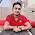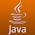This blog is under construction

Tuesday, 9 July 2013

C program to print even, odd and prime factors of a given number

Write a C program to print even, odd and prime factors of a given number.

#include <stdio.h>
#include <string.h>

/* tell whether the given number is even, odd or prime */
void numType(int data, char *type) {
int i, flag = 1;

/* checking whether the given no is prime */
for (i = 2; i <= data - 1; i++) {
if (data % i == 0) {
flag = 0;
break;
}
}

if (data != 1 && flag) {
strcpy(type, "a prime");
return;
}

/* check for even or odd */
if (data % 2 == 0) {
strcpy(type, "an even");
} else {
strcpy(type, "an odd");
}
return;
}

int main() {
int num, i;
char type;

/* get the input value from the user */
printf("Enter the value for n:");
scanf("%d", &num);

/* prints factors and its type */
for (i = 1; i <= num; i++) {
if (num % i == 0) {
numType(i, type);
printf("Factor %d is %s number\n", i, type);
}
}

return 0;
}

Output:
jp@jp-VirtualBox:~/\$ ./a.out
Enter the value for n:100
Factor 1 is an odd number
Factor 2 is a prime number
Factor 4 is an even number
Factor 5 is a prime number
Factor 10 is an even number
Factor 20 is an even number
Factor 25 is an odd number
Factor 50 is an even number
Factor 100 is an even number

1.C program to check odd or even

In general Even numbers are those which are divisible by 2, and which numbers are not divisible 2 is called odd numbers.
We can easily write even odd program in c programming.

2.Very Nice Post visit nice example also Even odd example in java

3.Visit nice example Even odd logic